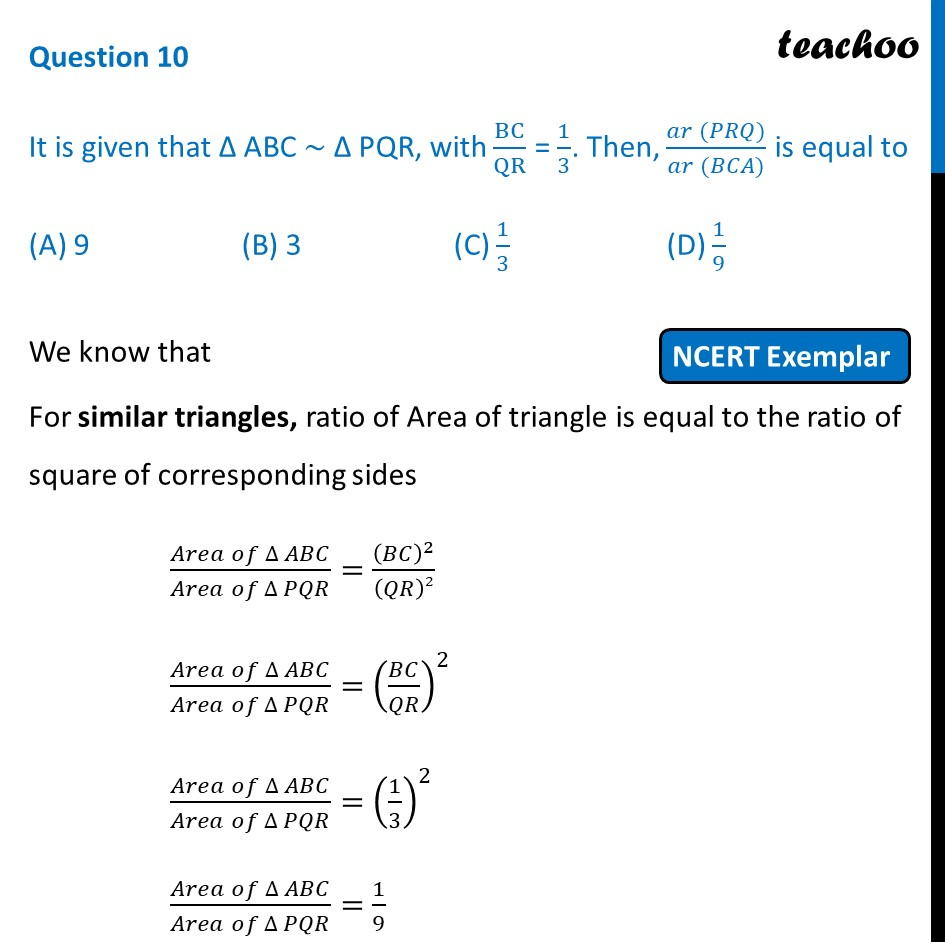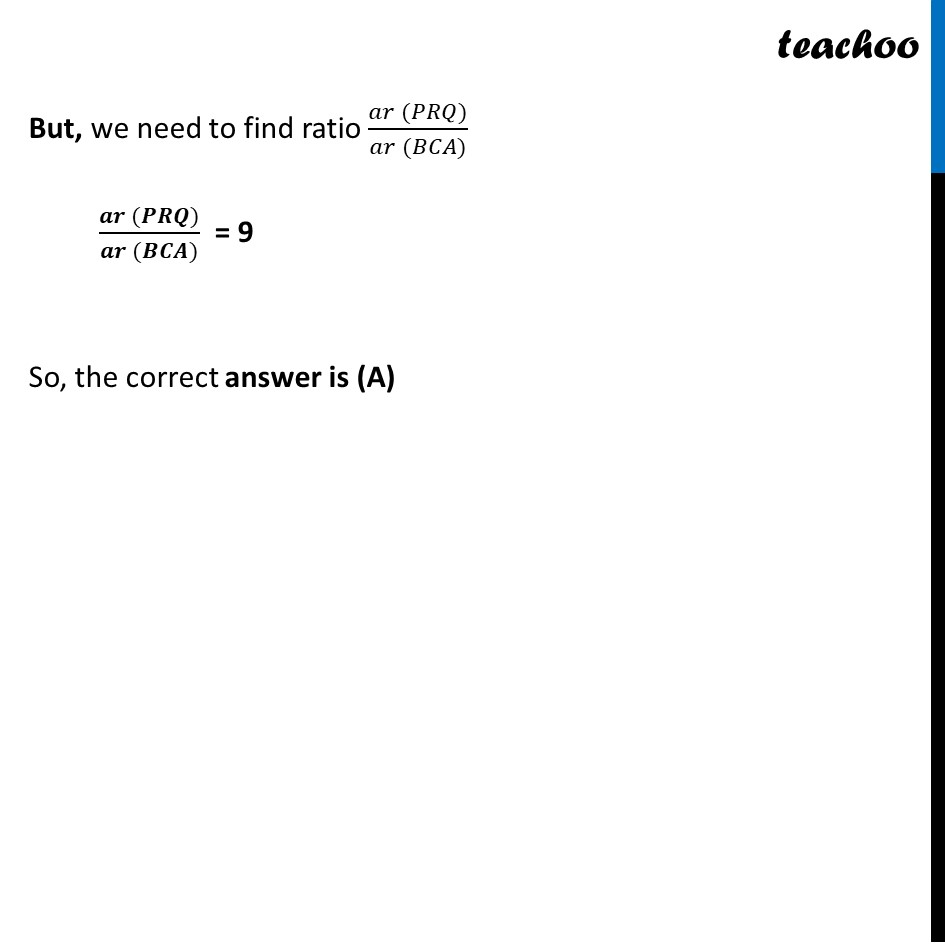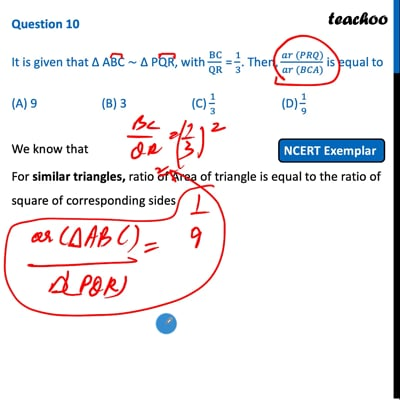NCERT Exemplar - MCQ

Chapter 6 Class 10 Triangles
Serial order wise

## (A) 9  (B) 3  (C) 1/3  (D) 1/9This video is only available for Teachoo black users

Maths Crash Course - Live lectures + all videos + Real time Doubt solving!

### Transcript

Question 10 It is given that ∆ ABC ~ ∆ PQR, with BC/QR = 1/3. Then, (𝑎𝑟 (𝑃𝑅𝑄))/(𝑎𝑟 (𝐵𝐶𝐴)) is equal to (A) 9 (B) 3 (C) 1/3 (D) 1/9 We know that For similar triangles, ratio of Area of triangle is equal to the ratio of square of corresponding sides (𝐴𝑟𝑒𝑎 𝑜𝑓 ∆ 𝐴𝐵𝐶)/(𝐴𝑟𝑒𝑎 𝑜𝑓 ∆ 𝑃𝑄𝑅)=(𝐵𝐶)^2/(𝑄𝑅)2 (𝐴𝑟𝑒𝑎 𝑜𝑓 ∆ 𝐴𝐵𝐶)/(𝐴𝑟𝑒𝑎 𝑜𝑓 ∆ 𝑃𝑄𝑅)=(𝐵𝐶/𝑄𝑅)^2 (𝐴𝑟𝑒𝑎 𝑜𝑓 ∆ 𝐴𝐵𝐶)/(𝐴𝑟𝑒𝑎 𝑜𝑓 ∆ 𝑃𝑄𝑅)=(1/3)^2 (𝐴𝑟𝑒𝑎 𝑜𝑓 ∆ 𝐴𝐵𝐶)/(𝐴𝑟𝑒𝑎 𝑜𝑓 ∆ 𝑃𝑄𝑅)=1/9 But, we need to find ratio (𝑎𝑟 (𝑃𝑅𝑄))/(𝑎𝑟 (𝐵𝐶𝐴)) (𝒂𝒓 (𝑷𝑹𝑸))/(𝒂𝒓 (𝑩𝑪𝑨)) = 9 So, the correct answer is (A)